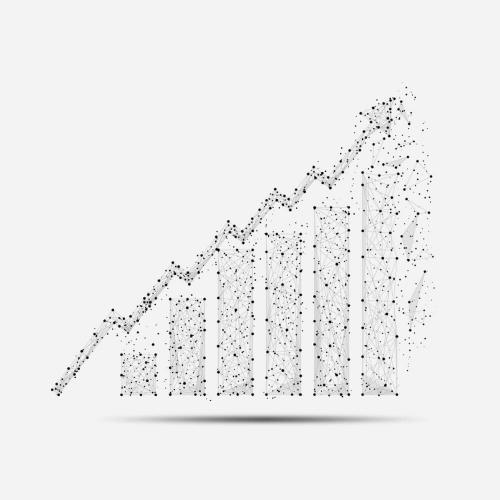# Time Series Analysis

Back to Programs## We created this program for those of you, who are interested in working with time series data.

During the first semester, we focus on the essential fundamentals, such as basic concepts of math and programming, which are the same for all programs.

In the Math & Statistic course, students will learn about discrete mathematics and the basics of probability theory. Algorithms & Data Structures covers the basic algorithms on graphs, data structures, and recurrence relations. Also, during the first semester, students learn how to process and analyze data, and build simple models based on this data in the Data Science course. In the Advanced Programming Languages course, students will learn about programming languages and their fundamental differences.

During the second semester, students will get acquainted with the basics of machine learning, learn how the main machine learning models work (in the Machine Learning course), and also try to use them in practice on complex tasks in the Data Science course (part 2). Students of this program will also take a Systems Architecture training course.

The major course of this program is Practical Time Series Analysis. We will offer students to put into practice their knowledge of algorithms for constructing and analyzing time-series data, including, but not limited to, analysis of various indicators of the financial markets.

## Semester 1

#### Math & Statistics

• Number theory

• Sets, functions, and sequences

• Generalized Mobius function and asymptotics

• Relations

• Recurrence relations

• Counting techniques

• Logic and techniques of proof

• Trees and graphs

• Partitioning numbers into terms

• Algorithms

• Mathematical theory of sampling

• Normal populations and distributions

• Chi-square, t, and F distributions

• Hypothesis testing

• Estimation

• Confidence intervals

• Sequential analysis

• Correlation, regression

• Analysis of variance

• Limit theorems

• Chromatic numbers of graphs and Kneser graph

#### Algorithms and Data Structures

• Simple data structures

• Sorting

• Binary and ternary search

• Divide and conquer method

• Recursion

• Hash functions and hash tables

• Graphs

• Dynamic programming

• Greedy algorithms

• Tree data structures

• Balanced trees

• Heap, priority queue, and more

• String algorithms

• Segment tree, Fenwick tree, SQRT-decomposition, Cartesian tree, and more

• Iterating over all subsets for som sets

• Optimizations

• Enumeration of all combinations, permutations, other combinations

#### Data Science

• Basic tools for data analysis

• Simple data collection and analysis

• Probability and expected values

• Variability, distribution, and asymptotics

• Intervals, testing, and Pvalues

• Power, bootstrapping, and permutation tests

• Logistic regression & poisson regression

• Prediction, errors, cross-validation

• Caret package

• Predicting with trees, random forests, and model-based predictions

• Regularized regression and combining predictions

• Exploratory data analysis & modeling

• Prediction model

• Creative exploration

• Least-squares, linear regression, multivariable regression, residuals, and diagnostics

• Language design principles

• Programming languages

• Syntax description

• Semantic description

• Lexical and syntax analysis

• Variables

• Data types and assignment statements

• Expressions and assignments

• Parallel and concurrent programming

• Functional programming

• Logic programming

## Semester 2

#### Machine Learning

• Introduction to ML and examples of basic tasks

• EM-algorithm and network of radial basis functions

• Metric and linear classification algorithms

• SVM

• Linear classification methods

• Regression recovery methods

• Neural networks

• Clustering algorithms

• Compositions of classifiers

• Boosting

• Logical classification algorithms

• Decision trees

• Weighted voting and more

• Bayesian classification algorithms, non-parametric methods, parametric methods, normal discriminant analysis

#### Systems Architecture

• System calls and interrupt handling

• The concept of process, stream, and thread

• Stream synchronization

• Classification of types of memory

• Pointers

• Process memory

• Disk storage device

• File systems

• Architecture: scheduler, memory manager, IPC

• Basic elements of the operating system

#### Data Science

• Practical application of the methods studied in the first half of the course

• Solving practical problems on regression and classification

• Familiarity with Tensorflow and Keras using examples of classical tasks

• Building neural networks and more

#### Practical Time Series Analysis

• Statistical models

• Time series graphics and visualization

• Stationary processes and features

• Time series decomposition

• Regression models

• Exponential smoothing

• Linear processes

• Non-stationary processes

• Counted time series

• Markov chains

• Adaptive selection and composition of forecasting models

• Examples of applied tasks: forecasting traffic, number of visits, sales volumes